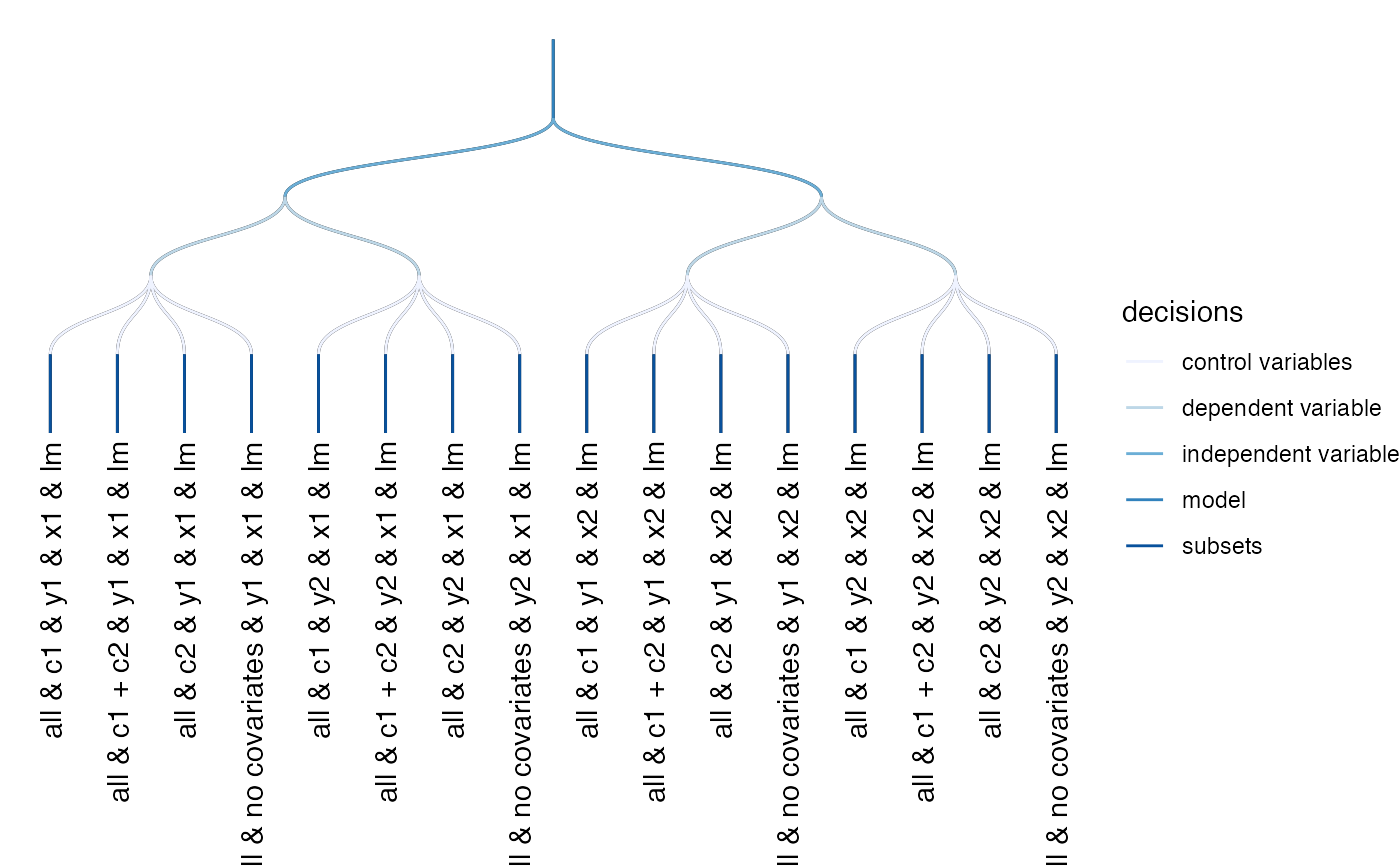This function plots a simple decision tree that is meant to help understanding how few analytical choices may results in a large number of specifications. It is somewhat useless if the final number of specifications is very high.

plot_decisiontree(df, label = FALSE, legend = FALSE)

## Arguments

df

data frame resulting from run_specs().

label

Logical. Should labels be included? Defaults to FALSE. Produces only a reasonable plot if number of specifications is low.

legend

Logical. Should specific decisions be identifiable. Defaults to FALSE.

a ggplot object.

## Examples

results <- run_specs(df = example_data,
y = c("y1", "y2"),
x = c("x1", "x2"),
model = c("lm"),
controls = c("c1", "c2"))

# Basic, non-labelled decisions tree
plot_decisiontree(results)# Labelled decisions tree
plot_decisiontree(results, label = TRUE)